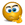# Countif, based on result calculated from values in multiple ranges

## Excel Help for Countif, Based On Result Calculated From Values In Multiple Ranges in Analyzing Corporate Financial DataI have two separate ranges on a spreadsheet. In range A5:A20, there are some numbers. In range H5:H20, there are other numbers. Suppose I did a calculation like this (for each row): =A5+(H5*0.6). Then further suppose that I wanted to countif the resulting value was greater than 38.5 - this is what I want to use the countif or countifs function to do. Of course, I could set up another range, say J5:J20, to perform the calculation above and then use this formula: =COUNTIF(J5:J20,">38.5"). However, I don't want such a column to appear. I would prefer to simply write a formula that can give me the answer in one hit. Any way to do it? Posted by Dave P on 21 Feb 2023